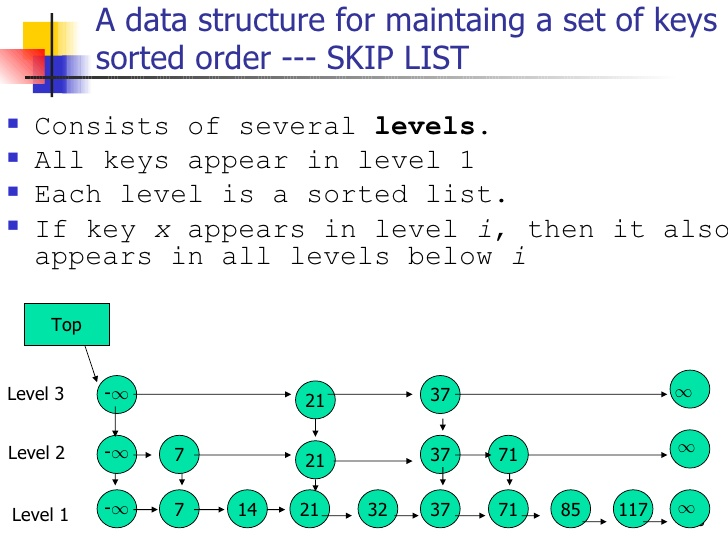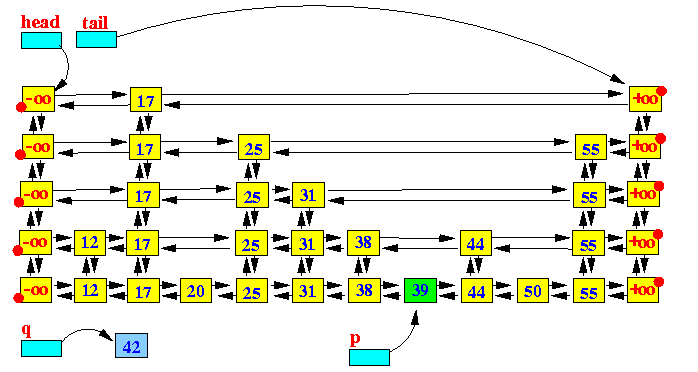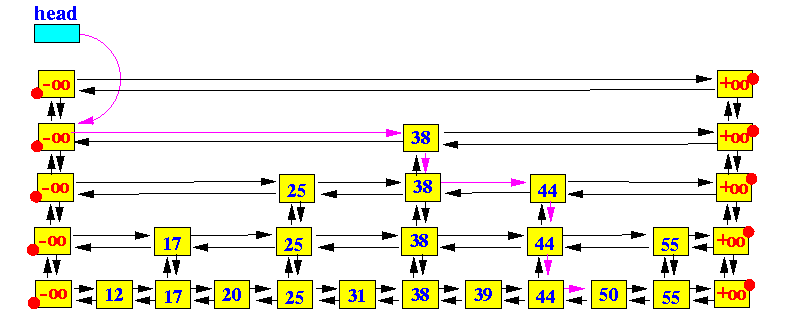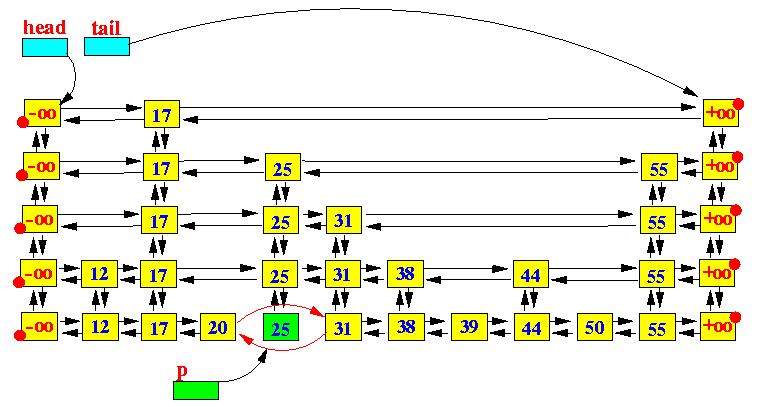# 1119. Data Structure - Skip ListSkipList

Implement SkipList.

## 1. Skip List

### 1.2 Problem of Linked List

A linked list is represented by a sequence of nodes. Each node contains a link to another node. The worst case search time for a sorted linked list is O(n) as we can only linearly traverse the list and cannot skip nodes while searching.

### 1.2 Skip List

A skip list is a data structure that allows log(n) search complexity as well as log(n) insertion complexity within an ordered sequence of n elements. It skips over many of the items of the full list in one step, that’s why it is known as skip list.### 1.3 Insert, Search and Delete

Insert 44.Search 44.Delete 25.## 2. Implementation

Define skip list node.

public class SkipNode {
public int val;

public SkipNode left;
public SkipNode right;
public SkipNode up;
public SkipNode down;

public SkipNode(int val) {
this.val = val;
this.left = null;
this.right = null;
this.up = null;
this.down = null;
}

public SkipNode(SkipNode lowerLevelNode) {
this.val = lowerLevelNode.val;
this.left = null;
this.right = null;
this.up = null;
this.down = lowerLevelNode;
}
}


List.

import java.util.ArrayList;
import java.util.List;

public class SkipList {
/*
* These are starting pointers. They are always from top layer.
*/
private SkipNode tail;

public SkipList() {
tail = new SkipNode(Integer.MAX_VALUE);

}

public SkipNode search(int val) {

while (curr != null) {
while (curr.right != null && curr.right.val <= val ) {
curr = curr.right;
}

if (curr.val == val) {
break;
}

curr = curr.down;
}

return curr;
}

public boolean insert(int data) {
List<SkipNode> pointersToUpdate = new ArrayList<>();

while (curr != null) {
while (curr.right != null && curr.right.val < data ) {
curr = curr.right;
}

curr = curr.down;
}

// insert after this node.
int level = 0;
SkipNode newNode = null;

while (level == 0 || flipCoin()) {
// now move up.
if (newNode == null) {
newNode = new SkipNode(data);
} else {
newNode = new SkipNode(newNode);
}

SkipNode nodeToUpdate;

if (pointersToUpdate.size() <= level) {
createNewLayer();
} else {
nodeToUpdate = pointersToUpdate.get(pointersToUpdate.size() - level - 1);
}

// insert
newNode.right = nodeToUpdate.right;
newNode.left = nodeToUpdate;

newNode.right.left = newNode;
nodeToUpdate.right = newNode;

level++;
}

return true;
}

public boolean delete(int data) {
List<SkipNode> pointersToUpdate = new ArrayList<>();

while (curr != null) {
while (curr.right != null && curr.right.val < data ) {
curr = curr.right;
}

if (curr.right.val == data) {
}

curr = curr.down;
}

for (int i = 0; i < pointersToUpdate.size(); i++) {
SkipNode nodeToUpdate = pointersToUpdate.get(i);
SkipNode nodeToDelete = nodeToUpdate.right;

nodeToUpdate.right = nodeToDelete.right;
nodeToDelete.right.left = nodeToUpdate;

nodeToDelete.up = null;
nodeToDelete.down = null;
}

return true;
}

private void createNewLayer() {
SkipNode newTail = new SkipNode(Integer.MAX_VALUE);

tail.up = newTail;
newTail.down = tail;
tail = newTail;
}

private boolean flipCoin() {
return Math.random() >= 0.5;
}

public void print() {

while (curr.down != null) {
curr = curr.down;
}

curr = curr.right;

while (curr.right != null) {
System.out.print(curr.val + " ");
curr = curr.right;
}

System.out.println();
}

public void printAllLevel() {

while (curr != null) {
SkipNode firstInLevel = curr;
firstInLevel = firstInLevel.right;

while (firstInLevel.right != null) {
System.out.print(firstInLevel.val + " ");
firstInLevel = firstInLevel.right;
}

curr = curr.down;
System.out.println();
}
}
}


Test.

public class SkipListExample {
public static void main( String[] args ) {
SkipList list = new SkipList();
list.insert(5);
list.insert(10);
list.insert(9);
list.insert(8);
list.insert(12);
list.insert(1);
list.insert(50);
list.insert(60);
list.insert(70);
list.insert(90);

list.print();
SkipNode node = list.search(9);
System.out.println(node.val);
//list.printAllLevel();

list.delete(10);
list.delete(1);
list.print();
//list.printAllLevel();
}
}


Output.

1 5 8 9 10 12 50 60 70 90
9
5 8 9 12 50 60 70 90